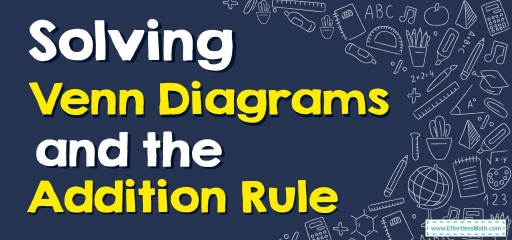# How to Solve Venn Diagrams and the Addition Rule?

The law of addition for probabilities describes two formulas, one for the probability of either of two mutually exclusive events happening and the other for the probability of two non-mutually exclusive events happening.A Venn diagram is a visual representation of the results of an investigation. It usually consists of a box containing the sample space $$S$$, as well as circles or ovals. The events are represented by circles or ovals.

## A step-by-step guide to Venn diagrams and the addition rule

In a probability experiment, if $$A$$ and $$B$$ are two occurrences, the likelihood that any of them will occur is:

$$\color{blue}{P (A\ or B)=P (A)+P (B)−P (A\ and B)}$$

The Venn diagram shows here as follows:

$$\color{blue}{P (A∪B)=P (A)+P (B)−P (A∩B)}$$

If $$A$$ and $$B$$ are two mutually exclusive events, $$P(A∩B)=0$$. The probability of either of the events occurring is then:

$$\color{blue}{P (A\ or B)=P (A)+P (B)}$$

In a Venn diagram, this might look like this:

$$\color{blue}{P(A∪B)=P(A)+P(B)}$$

### Venn Diagrams and the Addition Rule – Example 1:

In a group of $$103$$ students, $$32$$ are freshmen and $$46$$ are sophomores. Find the probability that a student picked from this group at random is either a freshman or sophomore.

First, find the probability of freshman and sophomore:

$$P( freshman)=\frac{32}{103}$$

$$P( sophomores)=\frac{46}{103}$$

Then, use this formula to find the probability of freshman or sophomore: $$\color{blue}{P (A\ or B)=P (A)+P (B)}$$

$$P( freshman\ or sophomore)=\frac{32}{103}+\frac{46}{103}=\frac{32+46}{103}=\frac{78}{103}$$.

### Venn Diagrams and the Addition Rule – Example 2:

In a group of $$114$$ students, $$41$$ are juniors, $$50$$ are male, and $$23$$ are male juniors. Find the probability that a student picked from this group at random is either a junior or male.

First, find the probability of juniors, male and male juniors:

$$P ( juniors)=\frac{41}{114}$$

$$P ( male)=\frac{50}{114}$$

$$P (male juniors)=\frac{23}{114}$$

Then, use this formula to find the probability junior or male: $$\color{blue}{P (A\ or B)=P (A)+P (B)−P (A\ and B)}$$

$$P( junior\ or male )=\frac{41}{114}+\frac{50}{114}-\frac{23}{114}=\frac{(41+50)-23}{114}=\frac{91-23}{114}=\frac{68}{114}$$

## Exercises for Venn Diagrams and the Addition Rule

1. We have to draw a card from a well-shuffled $$52$$-card deck. So what is the chance of getting a diamond or a face card?
2. For two events $$A$$ and $$B$$, $$P(A)= \frac{3}{5}$$, $$P(B) = \frac{3}{4}$$, and $$P(A∪B) = \frac{5}{6}$$. Find the probability of $$A∩B$$.
3. A glass jar consists of $$3$$ green,$$2$$ red, $$3$$ blue and $$4$$ yellow marbles. If a marble is randomly selected from a jar, how likely is it to be yellow or green?
4. A day of the week is chosen at random. What is the probability of choosing on Sunday or Monday?
1. $$\color{blue}{\frac{11}{26}}$$
2. $$\color{blue}{\frac{31}{60}}$$
3. $$\color{blue}{\frac{7}{12}}$$
4. $$\color{blue}{\frac{2}{7}}$$

### What people say about "How to Solve Venn Diagrams and the Addition Rule? - Effortless Math: We Help Students Learn to LOVE Mathematics"?

No one replied yet.

X
30% OFF

Limited time only!

Save Over 30%

SAVE $5 It was$16.99 now it is \$11.99